Next: Topic-specific PageRank Up: PageRank Previous: Definition:   Contents   Index

## The PageRank computation

How do we compute PageRank values? Recall the definition of a left eigenvector from Equation 214; the left eigenvectors of the transition probability matrix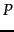are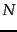-vectors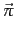such that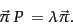(255)

Theentries in the principal eigenvectorare the steady-state probabilities of the random walk with teleporting, and thus the PageRank values for the corresponding web pages. We may interpret Equation 255 as follows: ifis the probability distribution of the surfer across the web pages, he remains in the steady-state distribution. Given thatis the steady-state distribution, we have that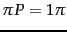, so 1 is an eigenvalue of P. Thus if we were to compute the principal left eigenvector of the matrix-- the one with eigenvalue 1 -- we would have computed the PageRank values.

There are many algorithms available for computing left eigenvectors; the references at the end of Chapter 18 and the present chapter are a guide to these. We give here a rather elementary method, sometimes known as power iteration. If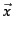is the initial distribution over the states, then the distribution at time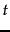is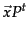. Asgrows large, we would expect that the distribution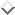is very similar to the distribution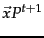, since for largewe would expect the Markov chain to attain its steady state. By Theorem 21.2.1 this is independent of the initial distribution. The power iteration method simulates the surfer's walk: begin at a state and run the walk for a large number of steps, keeping track of the visit frequencies for each of the states. After a large number of steps, these frequencies settle down'' so that the variation in the computed frequencies is below some predetermined threshold. We declare these tabulated frequencies to be the PageRank values.

We consider the web graph in Exercise 21.2.3 with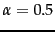. The transition probability matrix of the surfer's walk with teleportation is then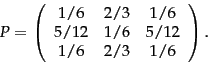(256)

Imagine that the surfer starts in state 1, corresponding to the initial probability distribution vector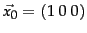. Then, after one step the distribution is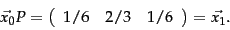(257)

After two steps it is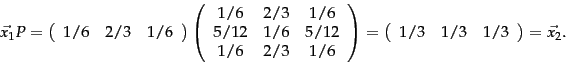(258)

Continuing in this fashion gives a sequence of probability vectors as shown in Figure 21.3 .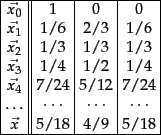Continuing for several steps, we see that the distribution converges to the steady state of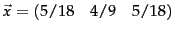. In this simple example, we may directly calculate this steady-state probability distribution by observing the symmetry of the Markov chain: states 1 and 3 are symmetric, as evident from the fact that the first and third rows of the transition probability matrix in Equation 256 are identical. Postulating, then, that they both have the same steady-state probability and denoting this probability by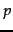, we know that the steady-state distribution is of the form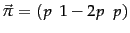. Now, using the identity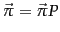, we solve a simple linear equation to obtain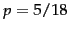and consequently,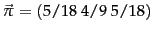.

The PageRank values of pages (and the implicit ordering amongst them) are independent of any query a user might pose; PageRank is thus a query-independent measure of the static quality of each web page (recall such static quality measures from Section 7.1.4 ). On the other hand, the relative ordering of pages should, intuitively, depend on the query being served. For this reason, search engines use static quality measures such as PageRank as just one of many factors in scoring a web page on a query. Indeed, the relative contribution of PageRank to the overall score may again be determined by machine-learned scoring as in Section 15.4.1 .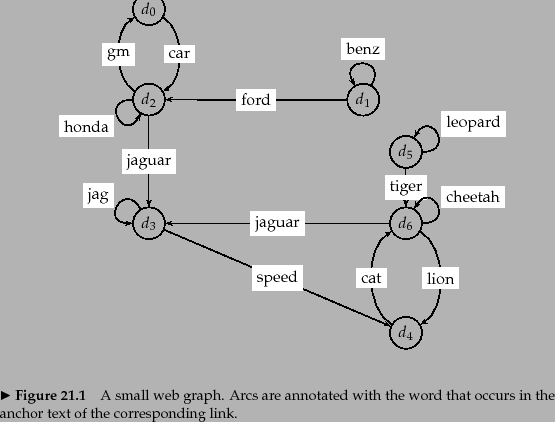Worked example. Consider the graph in Figure 21.4 . For a teleportation rate of 0.14 its (stochastic) transition probability matrix is: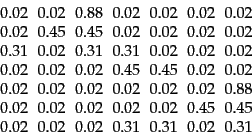(259)

The PageRank vector of this matrix is: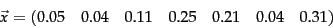(260)

Observe that in Figure 21.4 ,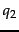,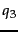,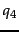and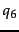are the nodes with at least two in-links. Of these,has the lowest PageRank since the random walk tends to drift out of the top part of the graph - the walker can only return there through teleportation. End worked example.Next: Topic-specific PageRank Up: PageRank Previous: Definition:   Contents   Index